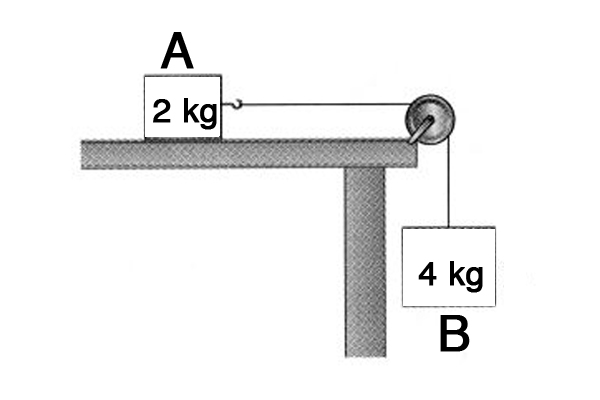# Motion on frictionless planeIn the above diagram, object $A$ is on a frictionless horizontal plane and is connected to object $B$ by a string and a pulley. What is the tension force on the string when both $A$ and $B$ start to move?

Gravitational acceleration is $g= 10 \text{ m/s}^2.$

×

Problem Loading...

Note Loading...

Set Loading...